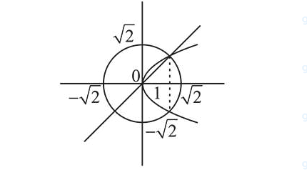# The area of the region, enclosed by the circle

Question:

The area of the region, enclosed by the circle $x^{2}+y^{2}=2$ which is not common to the region bounded by the parabola $y^{2}=x$ and the straight line $y=x$, is:

1. (1) $\frac{1}{6}(24 \pi-1)$

2. (2) $\frac{1}{3}(6 \pi-1)$

3. (3) $\frac{1}{3}(12 \pi-1)$

4. (4) $\frac{1}{6}(12 \pi-1)$

Correct Option: , 4

Solution:

Total area - enclosed area between line and parabola$=2 \pi-\int_{0}^{1} \sqrt{x}-x d x$

$=2 \pi-\left(\frac{2 x^{3 / 2}}{3}-\frac{x^{2}}{2}\right)_{0}^{1}$

$=2 \pi-\left(\frac{2}{3}-\frac{1}{2}\right)=2 \pi-\left(\frac{1}{6}\right)=\frac{12 \pi-1}{6}$